# Weld test - weld at centre of centrally-loaded simply-supported beam

## Introduction

This test answered a need to focus the most severe conditions in the sample upon the test weld being investigated. ie the test weld is the clear focus of the test procedure.

In a recent very interesting and rewarding learning exercise, the "U-frame RHS test" , a complimentary next test with this property would be needed to severely test welds and complete the range of information gained.

Considering the need, this test was visualised, which when implemented fulfilled that objective.

## The test

First-off - conveying an impression of how the tests were performed...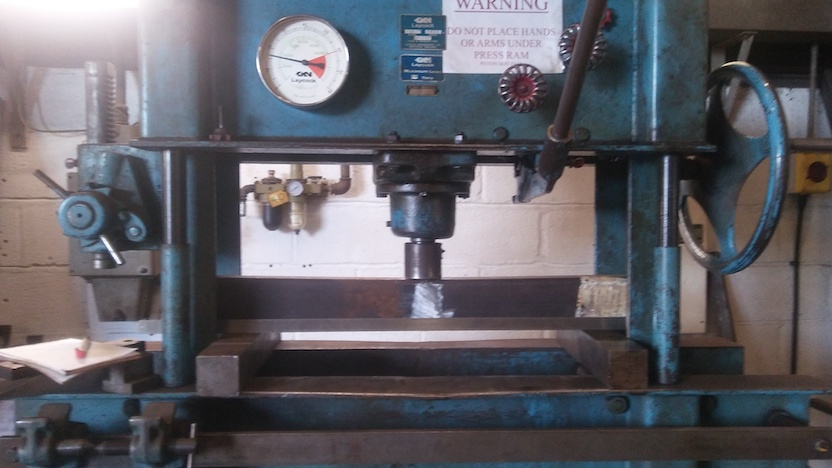Seen in overall picture of test setup:

• the press - blue-ish colour - higher specification version of those typical in metal fabrication shops and in garages (for pressing bearings, etc) - including gauge indicating force applied (Force=Pressure*Piston-area estimate)
• gauge on press is currently indicating about 16.5Tonnes-force
• the beam sample - seen under the cylindrical press tool - "silver" in middle where the test weld has been ground off flush on this side, and at right-hand-side end, otherwise with mill-scale on the Rectangular Hollow Section tube
• bearers - square solid steel, at 600mm apart = the gap between the bearers
• rule resting on the bearers part-obscuring the lower part of the sample in the picture - present to enable the extent of bending to be seen (given beam was initially straight)

Jump-ahead to immediately see effect of tests on the sample.

## Abbreviations applicable

• SHS - Structural Hollow Section
• RHS = Rectangular Hollow Section
• N = Newton - the SI unit of force
• kg-f = force, but expressed as if a weight borne, given Gravity=9.81N/kg on Earth
• Pa = Pascal - the SI unit of pressure and stress (N.m^-2)
• MPa = Mega-Pascals - millions of Pascals

## Presentation of further sections...

• Overall design of the test programme - the approach and reasons
• Details of the test
• The mathematics explained - application of Euler-Bernoulli beam theory
• The visible effects of the test on the welded beam sample
• Other observations
• Summary

## Overall design of the test programme - the approach and reasons

A straight beam supported at the ends and loaded in the middle has a highest stress at the mid-span.

What is meant here is a "simply supported beam" - which has an extensive agreed definition - and a point central loading.
Broadly, it you visualise a typical floor-joist in a house, the conditions meant are there.

Therefore

• get RHS steel stock of two equal lengths forming "half beams"
• your test weld butt-welds the two "half beams" coaxially into a "full beam"
• locate the beam ends on immovable bearers
• apply a single-point force in the middle
and the test weld is at the location of highest stress; therefore the focus of the test.

The "half beams" are compatible with the weld, but are less stressed, so the properties of the weld will dominate the outcome.

What is being done here is the exact opposite of what one would normally do being pragmatic when making a structure, which is to avoid welding near the middle of the beam. If it is necessary to butt beams together to make a longer length, put the longest stock length in the middle, and weld extensions towards the ends...

One attraction of this "simple" (?!) scheme with the sample as a straight beam is that the expected load-bearing ability and expected elastic deflection under load can be calculated with the Euler-Bernoulli beam equations.
That is - this situation has an "on-paper" arithmetic solution
(as contrasted with computer-numerical solutions ("number-crunching")).

Another fundamental attraction is that "axial" tensile tests require enormous applied forces and huge testing-machines - whereas beams can reach high stresses at moderate applied loads within reach of common general-purpose press.

## Details of the test

### The sample

• the "half beam" RHS's are (in mm.) 100x50by7.8, of "S355" (nominal 355MPa) steel
• the test weld is GMAW / MIG / MAG, with G3Si1 / ER70s-6 wire and Ar-CO2 shielding gas
• welding conditions are around 160A to 180A dip-transfer - around 18.5V to 20V
• single-V groove joint preparation; 30deg bevel -> 60deg included angle; 1mm root face
• root gap 1.6mm
• root run then at least 3 fill runs - to avoid excessive heat build-up in the weld zone
• "top" and one side of beam ground-off flush

### The set-up

The end supports for the test beam with test weld are set at 600mm gap between these supports.

## The mathematics explained - application of Euler-Bernoulli beam theory

*** mathematics not explained - study Second Moment of Area and Euler-Bernoulli beam equations if desired ***

To be calculated:

• applied load by press when the beam is predicted to (plastically) yield - the load beyond which at least part of the beam will permanent deform (be bent out of straight when at rest with zero load)
• the deflection of the beam at the load which causes onset of yielding
The predicted deflection at the moment of onset of yield did not prove particularly useful, as it is small and difficult to measure, and the load was easy to see on they press' force dial.

### Purely geometric initial stages

The Second Moment of Area, "I", of a square-cornered rectangle of the overall dimensions of the RHS = 2.443e-6 m^4
(using "bh^3/12" and subtracting 2ma-void from 2ma-outer)

Section Modulus, "Z" = 2I/h = 4.886e-5 m^3

### Bringing-in material property

Combining geometric properties with material properties to get service capability of this beam / RHS - the maximum Moment;
M_max=sigma_max.Z
M_max=355e6*4.886e-5
M_max=17347 Nm

### For the geometric situation of a simply-supported-beam

F_max=4.M_max/L
F_max=4*17347/0.6
F_max=115644 N

Converted - that is 11.8 Tonnes-force.

### Deflection of the test sample at the onset-of-yielding force

Deflection - for a simply-supported-beam
y=FL^3/48EI
Taking the elastic modulus as being 210GPa
y=F*0.6^3/(48*2.1e11*2.443e-6)
y=8.771e-9.F

From the calculations for force at onset of yielding, we have F_max=115644N
so at onset of yielding
y=1.014286e-03 m
or almost exactly 1mm.
Which is "small", in that "error" (in the strict scientific / experimental meaning) will easily overwhelm the attempted measurement.

### Euler-Bernoulli beam illustrated by FEA colourised output

An economical slightly naughty way of illustrating the under-load state of an Euler-Bernoulli beam is to grab the colourised renderings output of a Finite Element Analysis program modelling the beam.

This FEA model is very economical so is notably finely discretised, so the "exact" agreement between "exact" analytical equations and "approximate" FEA is a special case, be advised.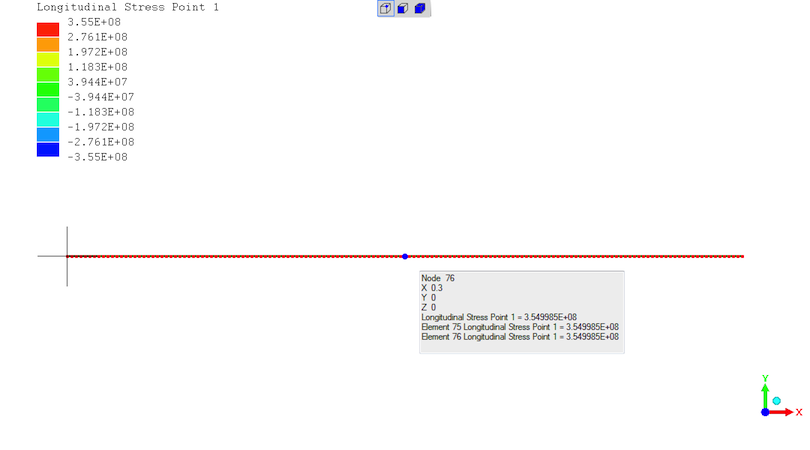Exact agreement on stress - "3.55E+08" = 355MPa at the midspan for a 115644N load.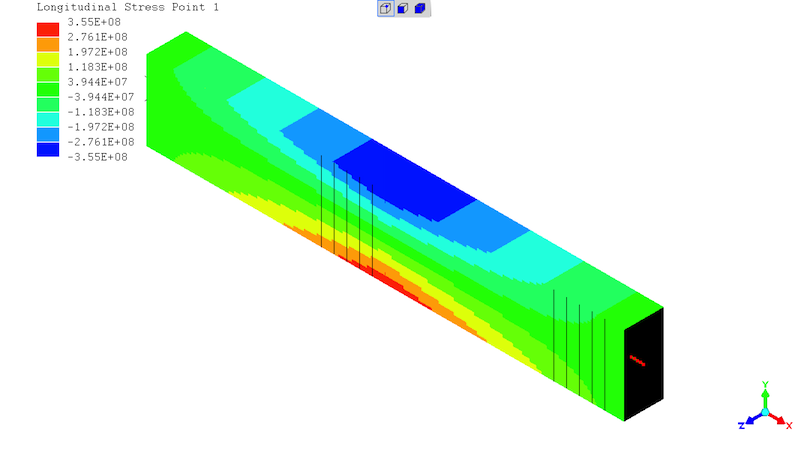(the 2 sets of 5 black lines are " rendering artifacts" with no real existence)
State of stresses in the beam - highest stresses at the mid-span with "negative" compression at the top and "positive" tension at the bottom.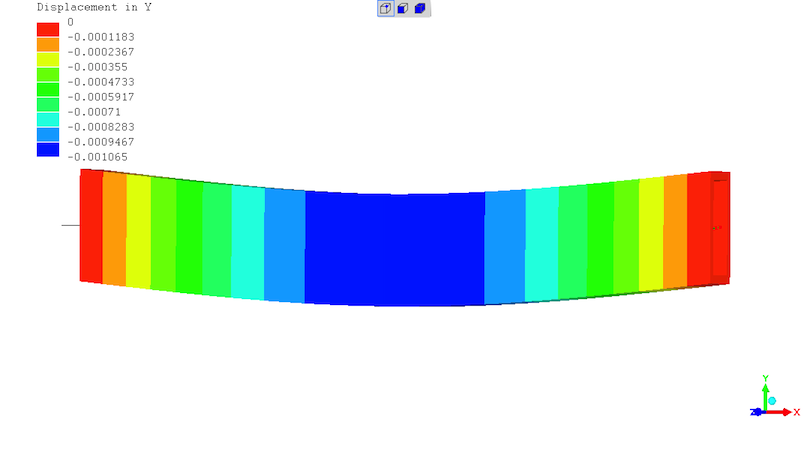Beam deflection off straight. In metres - so max. deflection is 1.065mm. Colourised and deflected shape represented at 20X magnification.

## The visible effects of the test on the welded beam sample

The test beam with test weld is shown when the press has been pumped-up to 17.6Tonnes-force, which is 1.5* the predicted force for the onset of yielding.
There being nothing obvious to picture at 11.8Tonnes-force of predicted onset of yielding.

The major "witness" to yielding are the Lu..ders (L u-umlaut d e r s) bands across the surface of the sample.

The Luders Bands have spalled-off the mill oxide coating on the RHS's, at angles to the beam axis tending to 45deg - which reasonable given shear is maximum on the 45deg inclinations.

In the last two photographs, bands are seen on the 45degree inclinations across the "silver" metal, supporting that these are Luders Bands.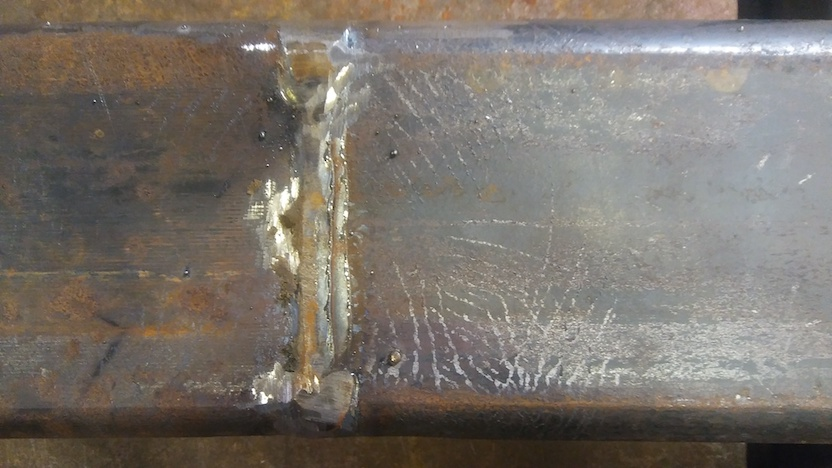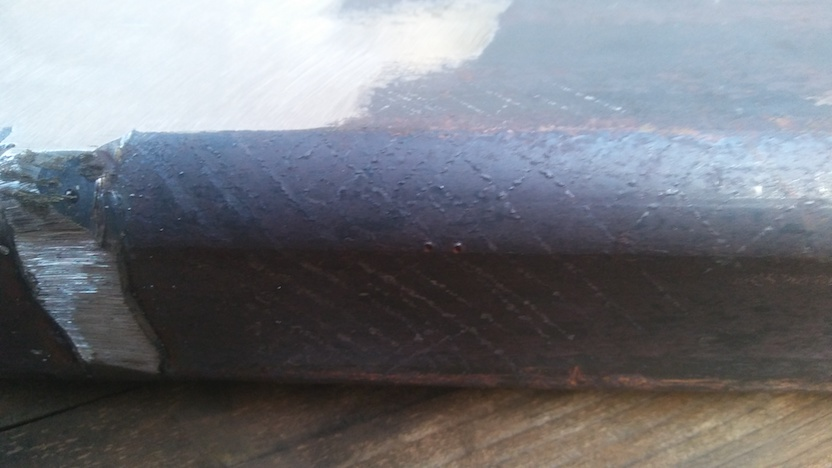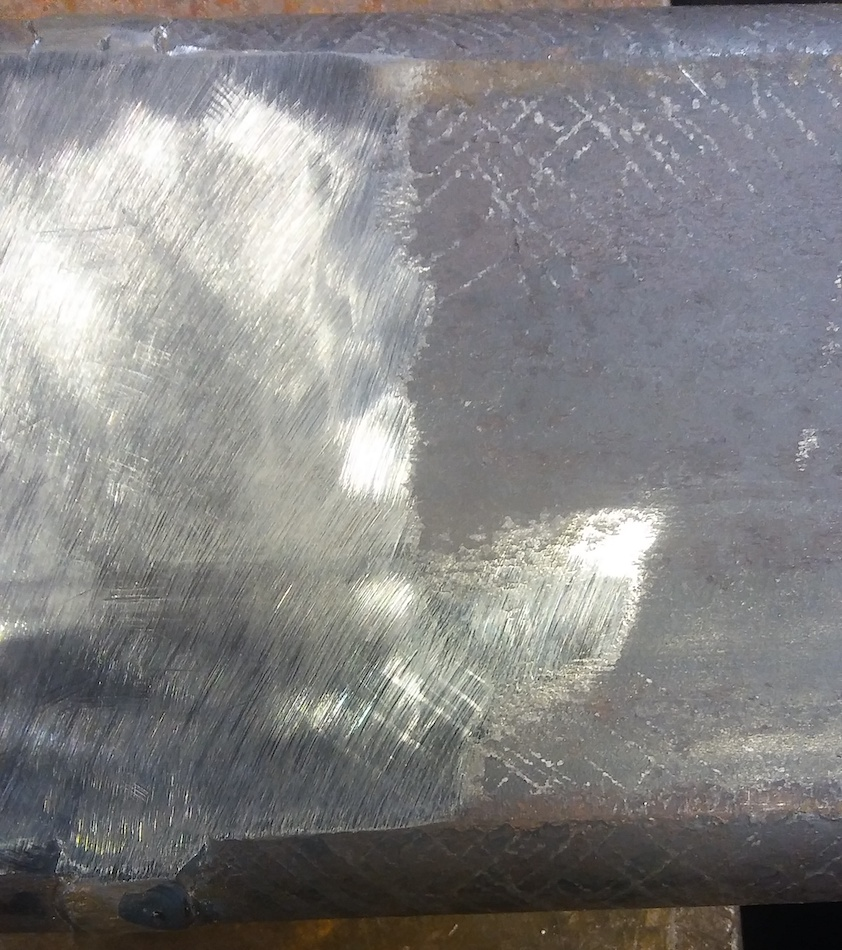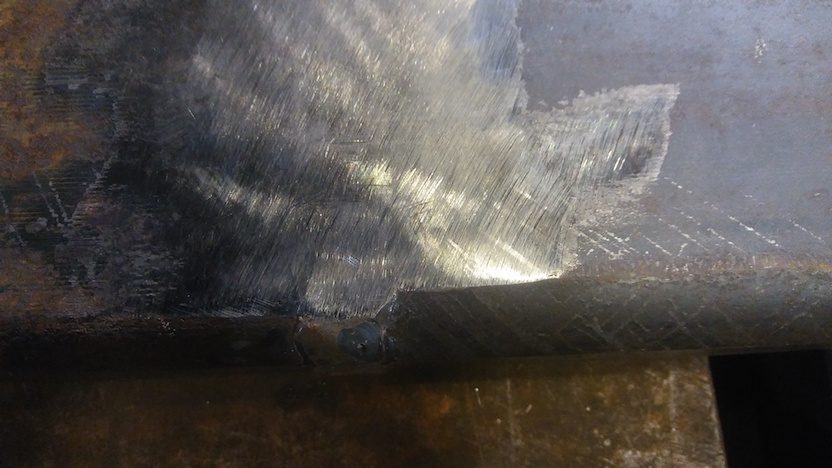The weld has no Luders Bands as can be observed - which is not surprising given the fine uniform structure of good welds. That said - the weld and the steel of the RHS are coupled, so the Luders Bands provide direct proof that the weld is being severely tested.
As intended...

## Other observations

As best as could be observed, and sensed through the lever of the hydraulic pump, the sample did reach yield at the predicted forces of 11.8Tonnes-force.
Luders Bands began appearing just over this force.

On releasing the press force, the beam maintained straightness up to 11.6Tonnes-force, but was visibly bent as seen when placed on a flat surface after more than 11.6Tonnes-force had been applied.

The deflection-under-load at 11.8Tonnes-force was measured as 1.5mm - which is indistinguishable from 1.0mm predicted given the likely experimental error.

At 17.6Tonnes-force, the measured deflection (certainly a mix of both elastic and plastic deformation) was 4.6mm. There is no expected value by Euler-Bernoulli theory after the onset of yielding is passed.

Wishing to recover the test beam for other uses, the beam was inverted and the press was pumped-up. Leading to the observation that the force which caused the bending (17.6Tonnes-force) was the force which returned the sample beam to straightness on releasing that load.
There was a large amount of movement at the same force, just approaching 11.6Tonnes-force, as judged by the number of strokes of the hydraulic pump with the force to pump remaining essentially constant.
There are complex metallurgic effects in elapsing time about yield-drop and recovery, which are probable here given there are Luders Bands, so it is not possible to say whether the effect is only observed if the force is reversed just after applying the force which caused the bending.

## Summary

This test worked very well indeed, for intended purpose.
Given the test was most testing of the weld's properties.
The materials measurement objectives were met, and the readily available equipment easily performed the tests.
The is abundant supporting evidence that the test is reliable and does what theory expects.
The Euler-Bernoulli equations do apply, making the test conditions easy to predict and the findings open to quantitative analysis.

(R. Smith, 24Nov2020, 29Nov2020 (added FEA beam images))